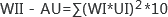微信授權賬號傳播指數WII-AU （V1.0）

（30%）

60%

15%

15%

10%

X1

X2

X3

X4

ln(X1+1)

ln(X2*1000+1)

ln(X3*10+1)

ln(X4*1000+1)

（10%）

60%

15%

15%

10%

X5

X6

X7

X8

ln(X5+1)

ln(X6*100+1)

ln(X7+1)

ln(X8*100+1)

（30%）

60%

15%

15%

10%

X9

X10

X11

X12

ln(X9+1)

ln(X10*1000+1)

ln(X11*100+1)

ln(X12*1000+1)

（30%）

15%

15%

20%

20%

30%

X13

X14

X15

X16

X17

ln(X13*100+1)

ln(X14+1)

ln(X15*100+1)

ln(X16+1)

ln(X17+1)